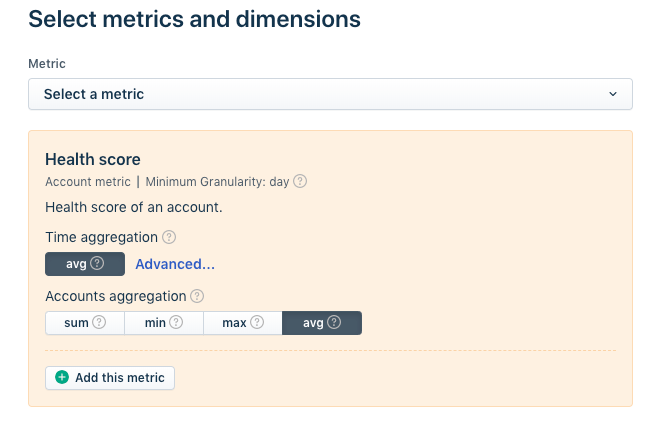### Overview

When creating a report in Freshsuccess chart explorer, you need to select a metric (or multiple metrics) to be plotted over time, how often (daily, monthly) that metric should be shown as well as the time frame over which that metric should be considered.

Since Freshsuccess aggregates metrics at a different frequency (indicated by Minimum Granularity), some daily, and some monthly and calculates them for each of your accounts, it is important to define what time aggregation and account aggregation you expect to see for a given metric, across a set of accounts and over a certain period of time.Depending on the minimum granularity of a selected metric, Time Aggregation asks you how you'd like Freshsuccess to aggregate that metric over the selected period for each account, and Account Aggregation asks you how you'd like Freshsuccess to further aggregate that number for a list of accounts you care about.

For each available aggregation type, it is calculated based on the following mechanism:

• Sum: Sum of the value across an account/user list relevant to the selected time granularity.

• Average: Average of the value across an account/user list relevant to the selected time granularity.

• Max: Max of the value by the account/user among the account/user list relevant to the selected time granularity.

• Min: Min of the value by the account/user among the account/user list over the selected time granularity.

• Count: Unique count of the value across an account/user list relevant to the selected time granularity.

### Example Calculation

For example, you have 3 accounts: A, B, C, with several days of data captured for a daily-granularity metric over the last month (30 days).

A: 5, 6, 3, 6

B: 2, 1, 4

C: 8, 9, 11

If the time aggregation is average, and the chart is plotted on a daily basis, below is how each account aggregation would look like:

• Sum: 15, 16, 18, 6

• Max: 8, 9, 11, 6

• Min: 2, 1, 3, 6

• Avg: (5+2+8)/3=5, (6+1+9)/3=5.3, (3+4+11)/3=6, 6/3=2

If time aggregation is average, and chart is plotted on a monthly basis, below is how each account aggregation would look like:

• Sum: (5+6+3+6)/30 + (2+1+4)/30 + (8+9+11)/30=1.83

• Max: Max[(5+6+3+6)/30, (2+1+4)/30, (8+9+11)/30]=0.93

• Min: Min[(5+6+3+6)/30, (2+1+4)/30, (8+9+11)/30]=0.23

• Avg: ((5+6+3+6)/30 + (2+1+4)/30 + (8+9+11)/30)/3=0.6

If time aggregation is sum, and chart is plotted on a daily basis, below is how each account aggregation would look like:

• Sum: 15, 16, 18, 6

• Max: 8, 9, 11, 6

• Min: 2, 1, 3, 6

• Avg: (5+2+8)/3=5, (6+1+9)/3=5.3, (3+4+11)/3=6, 6/3=2

If time aggregation is sum, and chart is plotted on a monthly basis, below is how each account aggregation would look like:

• Sum: 55

• Max: Max[(5+6+3+6),(2+1+4),(8+9+11)]=28

• Min: Min[(5+6+3+6),(2+1+4),(8+9+11)]=7

• Avg: ((5+6+3+6) + (2+1+4) + (8+9+11))/3 = 18.3